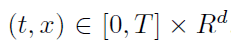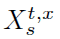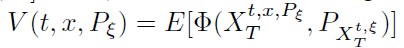Mean-field stochastic differential equations and associated PDEs
Speaker: Prof. Juan LIVice Dean of School of Mathematics and StatisticsShandong University 2 Feb 2015 (Monday) 11:00 - 12:00 E11-1009 Department of Mathematics

Abstract

In this paper we consider a mean-field stochastic differential equation, also called McKean- Vlasov equation, with initial  data,which coefficients depend on both the solutionbut also its law. We characterize the functionunder appropriate regularity conditions on the coefficients of the stochastic differential equation as the unique classical solution of a non local PDE of mean-field type, involving the first and second order derivatives of with respect to its space variable and the probability law. The proof bases heavily on a preliminary study of the first and second order derivatives of the solution of the mean-field stochastic differential equation with respect to the probability law and a corresponding Itˆo formula. The talk is based on a joint work with Rainer Buckdahn (UBO, Brest, France), Shige Peng (SDU, Jinan, PRC) and Catherine Rainer (UBO, Brest, France).

Biography

Professor Juan Li, vice dean of School of Mathematics and Statistics. She received the Ph.D. degree in probability and statistics from Shandong University, Jinan, China, in 2003. She has been a postdoctoral in Fudan University, China, and University of Brittany, France from 2005 to 2007. She joined Shandong University at Weihai, as an assistant professor in 1997, later she has been a full Professor since September 2007.

Her research interests include stochastic analysis, stochastic control theory, forward and backward stochastic differential equations and mathematical finance. Until now she has about 30 publications in high-rank international journals of mathematics, among them, Annals of Probability, SIAM Journal on Control and Optimization, Stochastic Processes and Their Applications.